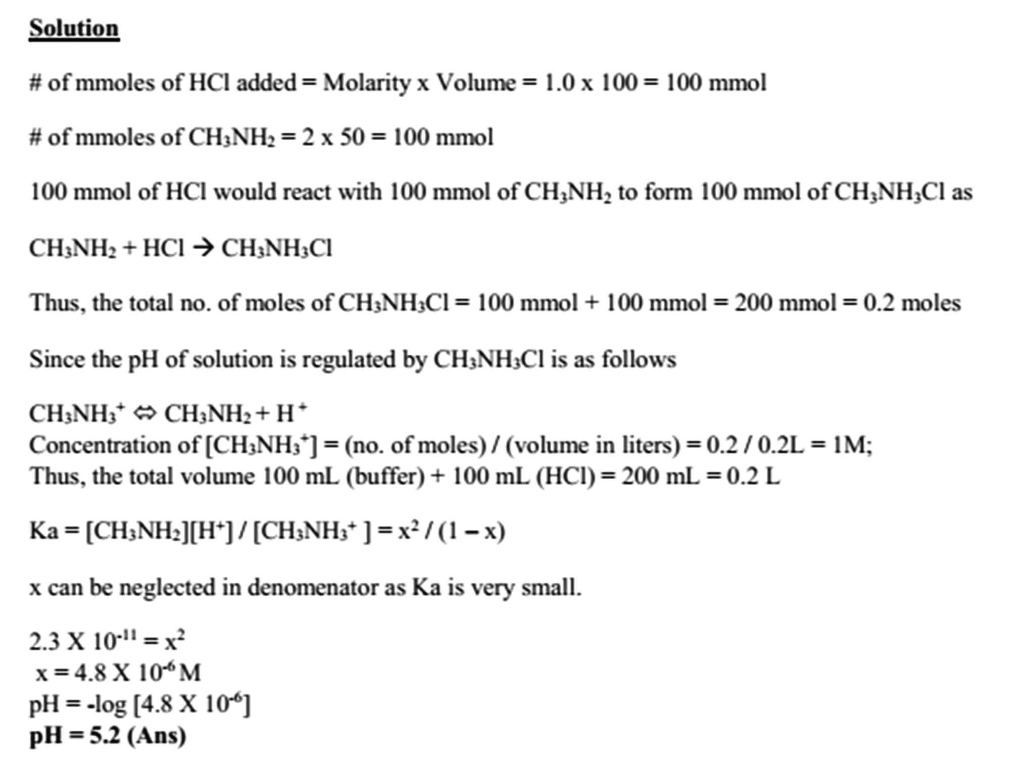In: Chemistry

# The following buffer was prepared: 50. mL 2.0 M methylamine (CH3NH2) solution and 50. mL of...

The following buffer was prepared: 50. mL 2.0 M methylamine (CH3NH2) solution and 50. mL of 2.0 M methylamine hydrochloride (CH3NH3Cl) solution. The Kb for methylamine is 4.4 x 10-4. What is the pH of the resulting solution after adding 100 mL of 1.0 M KOH to the original buffer solution?

## Solutions

##### Expert Solution## Related Solutions

##### The following buffer was prepared: 50. mL 2.0 M methylamine (CH3NH2) solution and 50. mL of...
The following buffer was prepared: 50. mL 2.0 M methylamine (CH3NH2) solution and 50. mL of 2.0 M methylamine hydrochloride (CH3NH3Cl) solution. The Kb for methylamine is 4.4 x 10-4. What is the pH of the resulting solution after adding 100 ml of 1.0 M HCl to the original buffer solution? a) 11.12 b) 10.64 c) 12.32 d) 0.48 e) 5.32
##### The following buffer was prepared: 50. mL 2.0 M methylamine (CH3NH2) solution and 50. mL of...
The following buffer was prepared: 50. mL 2.0 M methylamine (CH3NH2) solution and 50. mL of 2.0 M methylamine hydrochloride (CH3NH3Cl) solution. The Kb for methylamine is 4.4 x 10-4. A. What is the pH of the resulting solution after adding 200 ml of 1.0 M HCl to the original buffer solution? a)11.12                           b) 10.64                       c) 12.32                       d) 0.48                         e) 5.32 B.What is the pH of the resulting solution after adding 50 mL of 1.0 M KOH to the...
##### 50. mL 2.0 M methylamine (CH3NH2) solution and 50. mL of 2.0 M methylamine hydrochloride (CH3NH3Cl)...
50. mL 2.0 M methylamine (CH3NH2) solution and 50. mL of 2.0 M methylamine hydrochloride (CH3NH3Cl) solution. The Kb for methylamine is 4.4 x 10-4. What is the pH of the resulting solution after adding 100 mL of 1.0 M KOH to the original buffer solution? 11.12                           b) 10.64                       c) 12.32                       d) 0.48                         e) 5.32
##### 50 mL of 2.0 M NaOH is added to a 1.0L buffer solution that is 0.20M...
50 mL of 2.0 M NaOH is added to a 1.0L buffer solution that is 0.20M in HF and 0.20M in KF. The ka for HF is 3.5 x 10^-4. 1. Write the reaction or the buffer only including states and excluding spectator ions. 2. Write the neutralization reaction that occurs after the addition of LiOH including states and excluding spectator ions. 3. Calculate the amount of moles remaining and the final concentration for each component of the solution after...
##### What is the pH of a 0.360 M solution of Methylamine? Methylamine, CH3NH2, has a Kb...
What is the pH of a 0.360 M solution of Methylamine? Methylamine, CH3NH2, has a Kb = 4.40 x 10-4. Thank you!
##### Calculate the pH and concentrations of CH3NH2 and CH3NH3 in a 0.0395 M methylamine (CH3NH2) solution....
Calculate the pH and concentrations of CH3NH2 and CH3NH3 in a 0.0395 M methylamine (CH3NH2) solution. The Kb of CH3NH2 = 4.47 × 10-4.
##### A buffer solution was prepared by mixing 300.0 mL of a 0.200 M solution of hydroxylamine...
A buffer solution was prepared by mixing 300.0 mL of a 0.200 M solution of hydroxylamine (OHNH2, Kb = 1.1 × 10-8) and 250.0 mL of a 0.300 M solution of its hydrochloride salt (OHNH3+ Cl- ). Calculate the pH of the solution after the addition of 1.08 g of solid NaOH (molar mass = 40.00 g/mol). Assume that there is no volume change upon the addition of solid. A. 8.22    B. 5.55 C. 6.30 D. 5.78
##### A buffer solution was prepared by mixing 300.0 mL of a 0.200 M solution of hydroxylamine...
A buffer solution was prepared by mixing 300.0 mL of a 0.200 M solution of hydroxylamine (OHNH2, Kb = 1.1 × 10-8) and 250.0 mL of a 0.300 M solution of its hydrochloride salt (OHNH3+ Cl- ). Calculate the pH of the solution after the addition of 1.08 g of solid NaOH (molar mass = 40.00 g/mol). Assume that there is no volume change upon the addition of solid.
##### A buffer is prepared by dissolving some NaH2PO4 in 50 ml of a 0.2513 M NaOH...
A buffer is prepared by dissolving some NaH2PO4 in 50 ml of a 0.2513 M NaOH and diluting to a total volume of 100 ml. How many grams of NaH2PO4 are needed to give a buffer with a pH of 7.40? (MW NaH2PO4= 119.9772 g/mol)
##### A 230.0 −mL buffer solution initially contains 2.0×10−2 M of HCHO2 and 2.0×10−2 M of NaCHO2....
A 230.0 −mL buffer solution initially contains 2.0×10−2 M of HCHO2 and 2.0×10−2 M of NaCHO2. What mass of the correct reagent should you add?